# Dividing Fractions

Dividing Fractions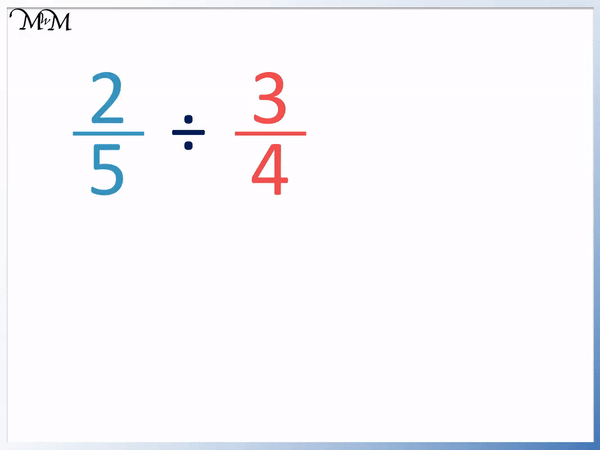• To divide a fraction by another fraction turn the division into a multiplication calculation.
• If we turn the division sign into a multiplication sign, we need to flip the fraction we are dividing by.
• We keep the first fraction the same.
• We flip the fraction we are dividing by.
•   2 / 5   ÷   3 / 4   becomes   2 / 5   ×   4 / 3  .
• We then can multiply the and the separately.
• The numerator in our answer is: 2 × 4 = 8.
• The denominator in our answer is: 5 × 3 = 15.
•   2 / 5   ÷   3 / 3   =   8 / 15  .

Write the division as a multiplication by flipping the fraction you are dividing by.

Then multiply the numerators and the denominators separately.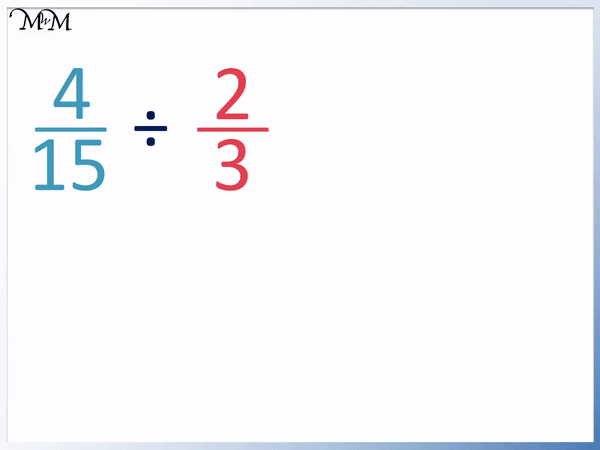• We keep the first fraction of   4 / 15   , we turn the division into a multiplication and we flip

2 / 3   to make it   3 / 2  .

• Multiplying the numerators we have 4 × 3 = 12.
• Multiplying the denominators we have 15 × 2 = 30.
• We can simplify   12 / 30   by dividing both digits by 6.
• The answer is   2 / 5  .
• We can see that in this question we can divide the numerators and denominators of the fraction immediately.
• 4 ÷ 2 = 2 and 15 ÷ 3 = 5.#### Dividing Fractions Interactive Question Generator

Dividing Fractions: Interactive Questions

# How to Divide Fractions

To divide fractions, it is very important to know how to multiply fractions first. We then turn the fraction division into a fraction multiplication so that we can work it out.

The method for dividing fractions is to keep the first fraction the same, turn the division into a multiplication and flip the fraction you are dividing by.

The rules for dividing fractions by fractions are shown in these steps:

1. Keep the first fraction the same
3. Flip the fraction you are dividing by upside down (write its reciprocal).
4. Multiply the numerators (tops) of the two fractions to form the numerator of the answer
5. Multiply the denominator (bottoms) of the two fractions to form the denominator of the answer

Division is the opposite of multiplication.

To divide by a fraction, we can turn the division into a multiplication if we flip the fraction we are dividing by upside down.The fraction of   3 / 4   means 3 ÷ 4.

The opposite of this is 4 ÷ 3, which can be written as the fraction   4 / 3   .

÷   3 / 4   is the same as   ×   4 / 3  .

We can see that we simply flip the fraction that we are dividing by.

Here is an example of   2 / 5   ÷   3 / 4  .When teaching dividing fractions, it is useful to write the division calculation as a multiplication below the original.

We keep the first fraction the same and so, we write   2 / 5   below.

We turn the division into a multiplication.

Because we did this, we flip the fraction we are dividing by.   3 / 4   becomes   4 / 3  .The fraction division is now written as a multiplication and so we can multiply to work out our answer.

We multiply the two numerators, which are the numbers on the top. These give us the numerator on the top of our answer.

2 × 4 = 8

We then multiply the denominators, which are the numbers on the bottom. These give us the denominator on the bottom of our answer.

5 × 3 = 15

2 / 5   ÷   3 / 4   =   8 / 15

In this next example of dividing fractions, we have   3 / 10   ÷   2 / 3  .

We use the method for dividing fractions of keep, multiply and flip..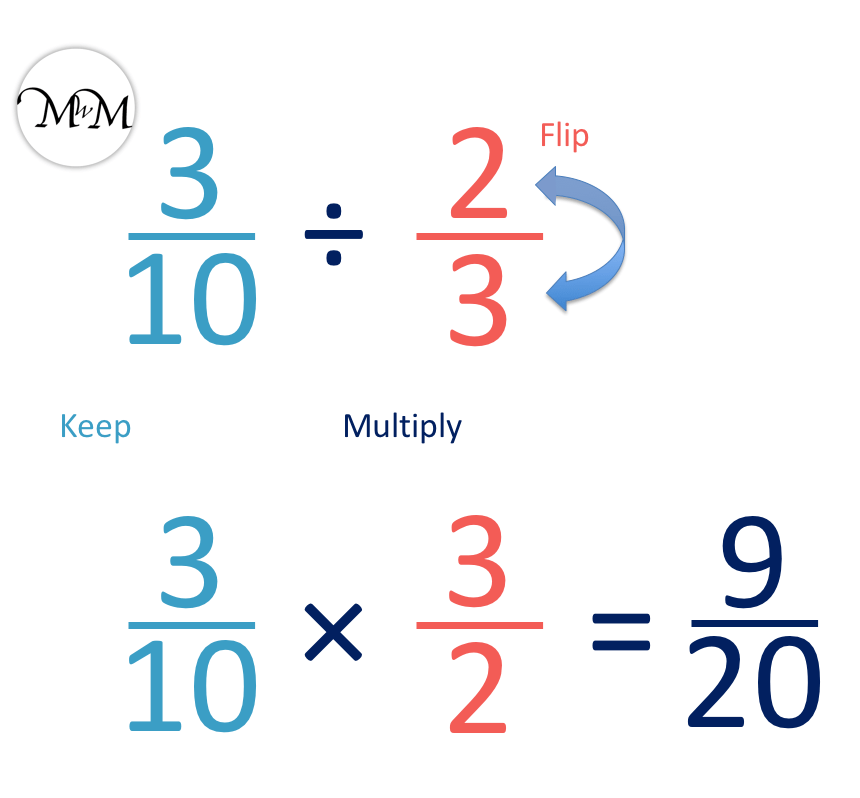We keep the first fraction of   3 / 10  .

Because we did this, we flip the second fraction.   2 / 3   becomes   3 / 2  .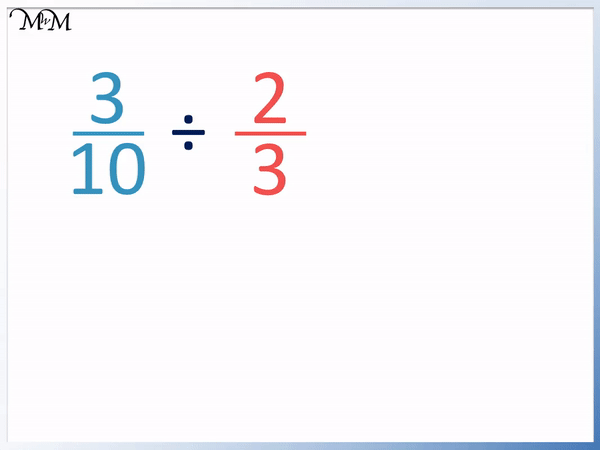We have turned the division of   3 / 10   ÷   2 / 3   into the multiplication of the fractions   3 / 10   ×   3 / 2  .

Multiplying the numerators we have 3 × 3 = 9.

Multiplying the denominators we have 10 × 2 = 20.

3 / 10   ÷   2 / 3   =   9 / 20  .

In this next dividing fractions example we have   3 / 8   ÷   1 / 2  .

We keep   3 / 8  .

We multiply.

We flip the fraction   1 / 2   to make   2 / 1  .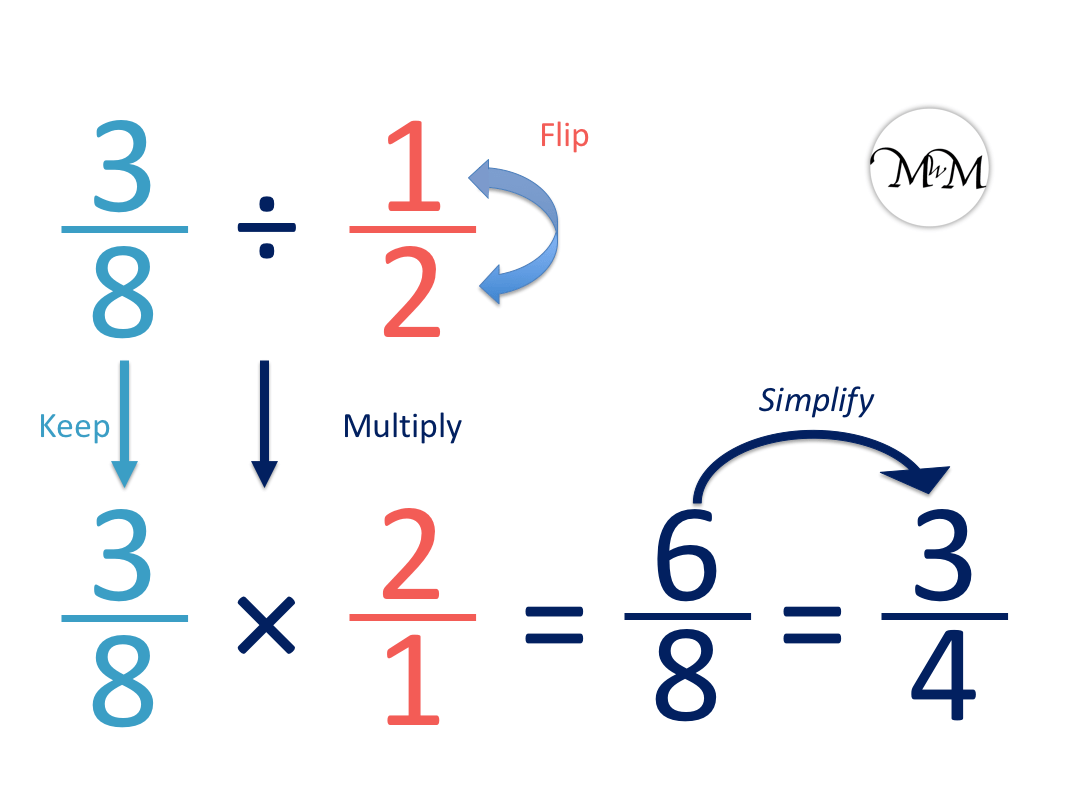We can multiply the numerators and denominators to get   6 / 8  .

When doing any fraction calculations, we try and simplify our answers wherever possible.

6 / 8   is made of the digits 6 and 8, which are both even.

We can divide both 6 and 8 by two to simplify the fraction.

6 ÷ 2 = 3 and 8 ÷ 2 = 4.

6 / 8   can be simplified to   3 / 4  .

3 / 8   ÷   1 / 2   =   3 / 4  .

Try to simplify all fractions that can be simplified when they are written as the final answer.We can notice a trick to help us do this division more easily.

If we can divide the numerator of the first fraction by the numerator of the second fraction exactly and we can divide the denominator of the first fraction by the denominator of the second fraction exactly, then we can do this to get our answer.

We see that in the original division question of   3 / 8   ÷   1 / 2  , we can see that 3 ÷ 1 = 3 and 8 ÷ 2 = 4.

The division on the top of the fractions gives us 3 and the division on the bottom of the fractions gives us 4. The answer is the same as we got to using the keep, multiply and flip method earlier. We get   3 / 4  .

Simply dividing the numerators and the denominators is much easier, however we can only do this with some questions where there is an exact division on both the top and bottom of the fraction. If we do not have this, then we use the method of keep, multiply and flip.

In this next example we have   4 / 15   ÷   2 / 3  .

We keep the first fraction of   4 / 15   the same.

We turn the division into a multiplication.

We flip the second fraction of 2 / 3   to produce 3 / 2  .We can now multiply the tops and bottoms of the two fractions separately to obtain the final answer.

4 × 3 = 12 and 15 × 2 = 30

The answer to   4 / 15   ÷   2 / 3   is   12 / 30  .Again in this example, we can see that this final answer can be simplified.

Both 12 and 30 are in the 6 times table and so, both numbers can be divided by 6.

12 ÷ 6 = 2 and 30 ÷ 6 = 5.

12 / 30   can be simplified to   2 / 5  .

Again in the original question of   4 / 15   ÷   2 / 3   , we can see that we have an exact division on the tops of the two fractions and an exact division on the bottoms.

Looking only at the numerators of the two fractions:

4 ÷ 2 = 2

and looking only at the denominators of the two fractions:

15 ÷ 3 = 5

4 / 15   ÷   2 / 3   =   2 / 5  . This is the same result that we obtained earlier.Now try our lesson on Converting Fractions to Percentages where we learn how to write a fraction as a percentage.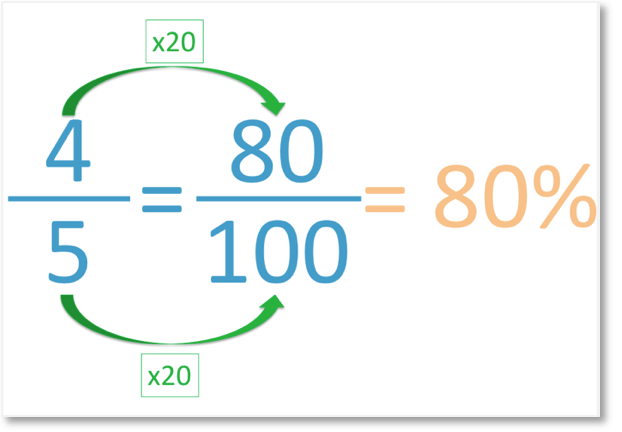error: Content is protected !!